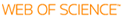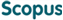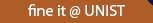### BROWSE

Numerical Methods for Hyperbolic Partial Differential Equations

Cited 0 times inCited 0 times inTitle
Numerical Methods for Hyperbolic Partial Differential Equations
Author
Keywords
Numerical Methods; WENO; Stiff Problems; Singular Perturbation; Polynomial Chaos Expansions
Issue Date
2015-08
Publisher
Abstract
In this dissertation, new numerical methods are proposed for different types of hyperbolic partial differential equations (PDEs). The objectives of these developments aim for the improvements in accuracy, robustness, efficiency, and reduction of the computational cost. The dissertation consists of two parts. The first half discusses shock-capturing methods for nonlinear hyperbolic conservation laws, and proposes a new adaptive weighted essentially non-oscillatory WENO-θ scheme in the context of finite difference. Depending on the smoothness of the large stencils used in the reconstruction of the numerical flux, a parameter θ is set adaptively to switch the scheme between a 5th-order upwind or 6th-order central discretization. A new indicator depending on parameter θ measures the smoothness of the large stencils in order to choose a smoother one for the reconstruction procedure. τ is devised based on the possible highest-order variations of the reconstructing polynomials in an L2 sense. In addition, a new set of smoothness indicators β_k’s of the sub-stencils is introduced. These are constructed in a central sense with respect to the Taylor expansions around point x_j . Numerical results show that the new scheme combines good properties of both 5th-order upwind and 6th-order central schemes. In particular, the new scheme captures discontinuities and resolves small-scaled structures much better than other 5th-order schemes; overcomes the loss of resolution near some critical regions; and is able to maintain symmetry which are drawbacks detected in other 6th-order central WENO schemes. The second part extends the scope to hyperbolic PDEs with uncertainty, and semi-analytical methods using singular perturbation analysis for dispersive PDEs. For the former, a hybrid operator splitting method is developed for computation of the two-dimensional transverse magnetic Maxwell equations in media with multiple random interfaces. By projecting the solutions into random space using the Polynomial Chaos (PC) expansions, the deterministic and random parts of the solution are solved separately. The deterministic parts are then numerically approximated by the FDTD method with domain decomposition implemented on a staggered grid. Statistic quantities are obtained by the Monte Carlo sampling in the post-processing stage. Parallel computing is proposed for which the computational cost grows linearly with the number of random interfaces. The last section deals with spectral methods for dispersive PDEs. The Kortewegde Vries (KdV) equation is chosen as a prototype. By Fourier series, the PDE is transformed into a system of ODEs which is stiff, that is, there are rapid oscillatory modes for large wavenumbers. A new semi-analytical method is proposed to tackle the difficulty. The new method is based on the classical integrating factor (IF) and exponential time differencing (ETD) schemes. The idea is to approximate analytically the stiff parts by the so-called correctors and numerically the non-stiff parts by the IF and ETD methods. It turns out that rapid oscillations are well absorbed by our corrector method, yielding better accuracy in the numerical results. Due to the nonlinearity, all Fourier modes interact with each other, causing the computation of the correctors to be very costly. In order to overcome this, the correctors are recursively constructed to accurately capture the stiffness of the mode interactions.
Description
Department of Mathematical Sciences
URIcan give you direct access to the published full text of this article. (UNISTARs only)